# RS Aggarwal Solutions for Class 8 Maths Chapter 3 - Square and Square Roots Exercise 3E

Students can download the RS Aggarwal Solutions for the Exercise 3E of Class 8 Maths Chapter 3, Squares and Square Roots from the link accessible here. BYJU’S masters have solved the questions present in the Exercise 3E in Maths, and this will help students in solving this exercise without any dilemmas. By practising regularly, the students can obtain reliable results in the Class 8 exams by using the RS Aggarwal Solutions. This exercise helps students to understand the concept of finding the square root of a perfect square by the long-division method.

By practising the RS Aggarwal Solutions for class 8, students will be able to grasp the concepts correctly. It also helps in raising their confidence, which plays a vital role in their examinations.

## Download PDF of RS Aggarwal Solutions for Class 8 Maths Chapter 3 – Squares and Square Roots – Exercise 3E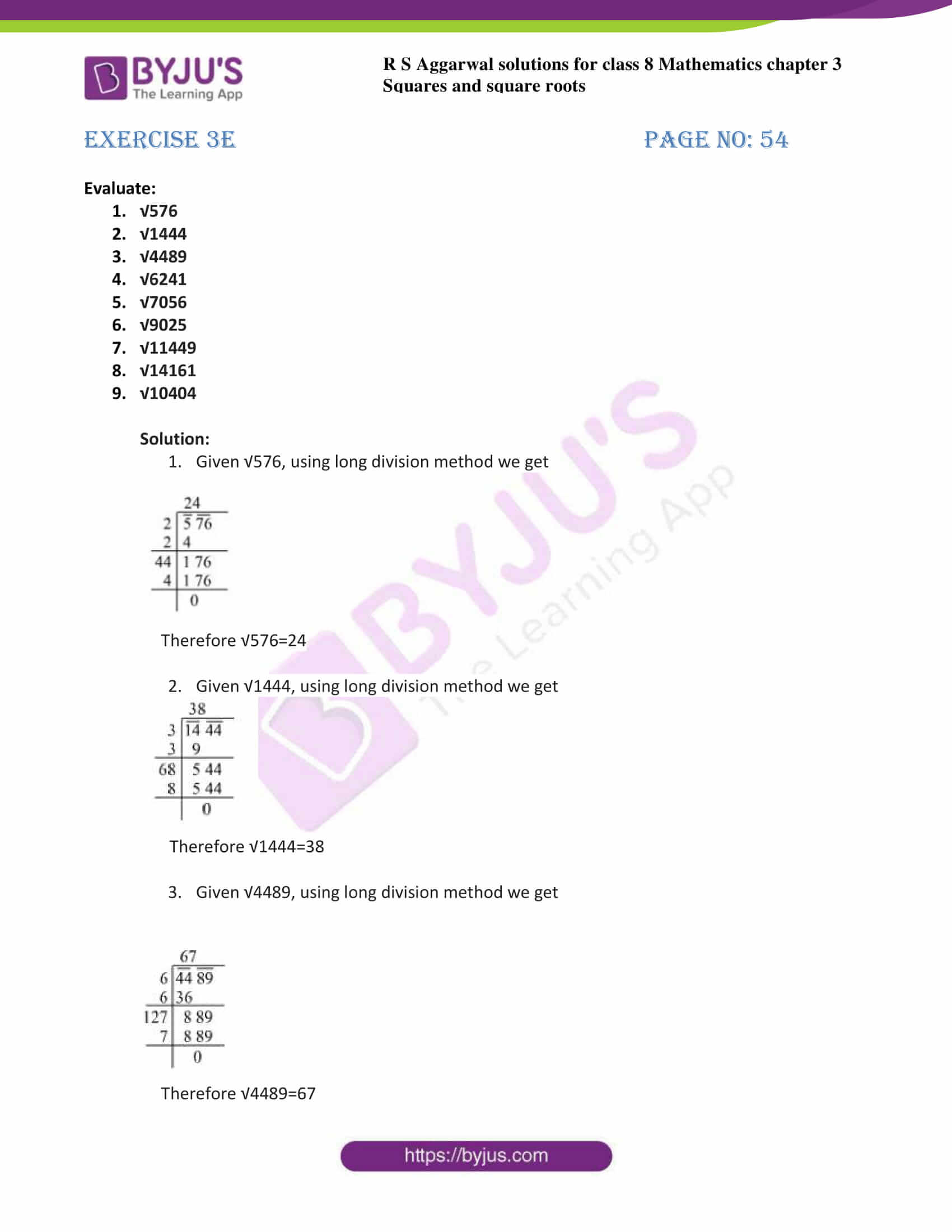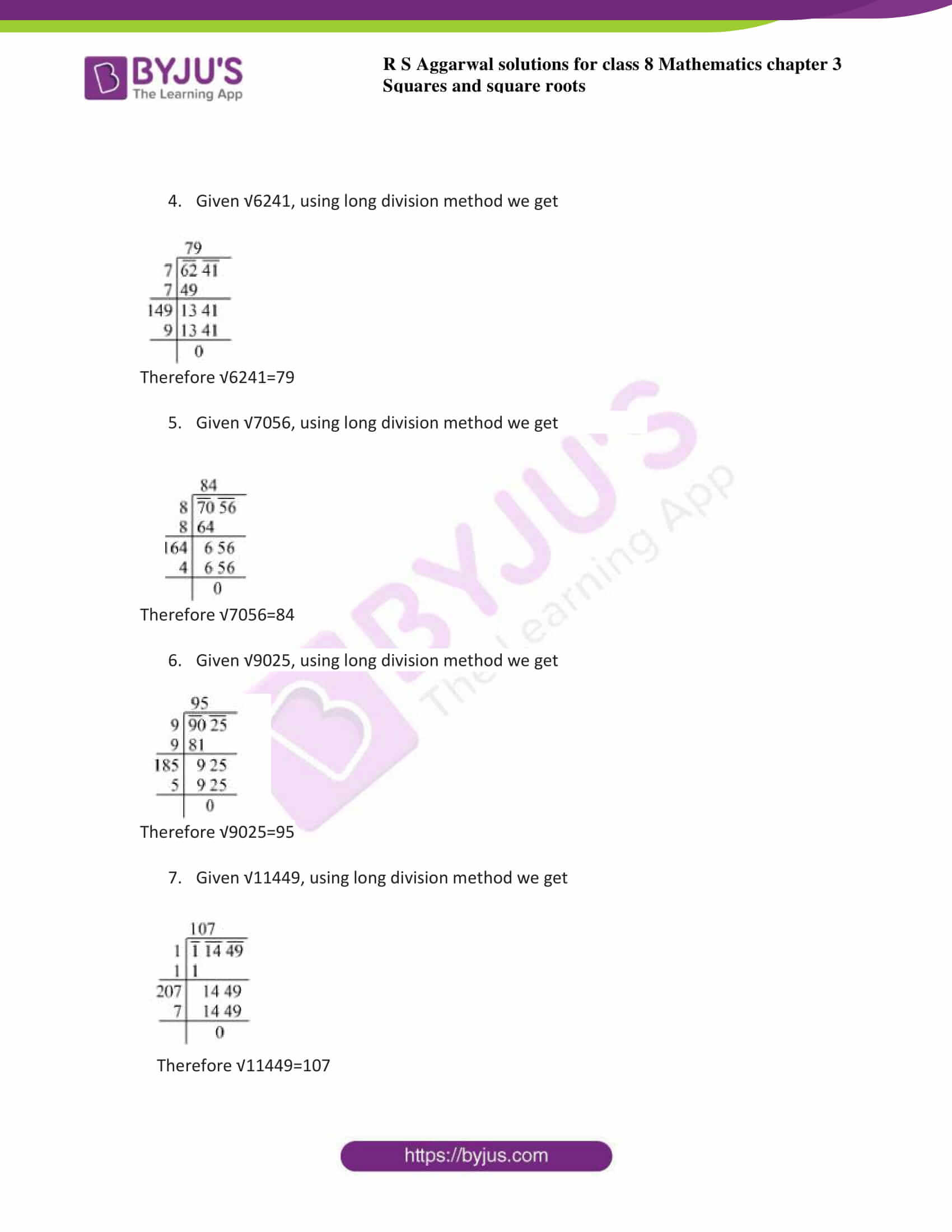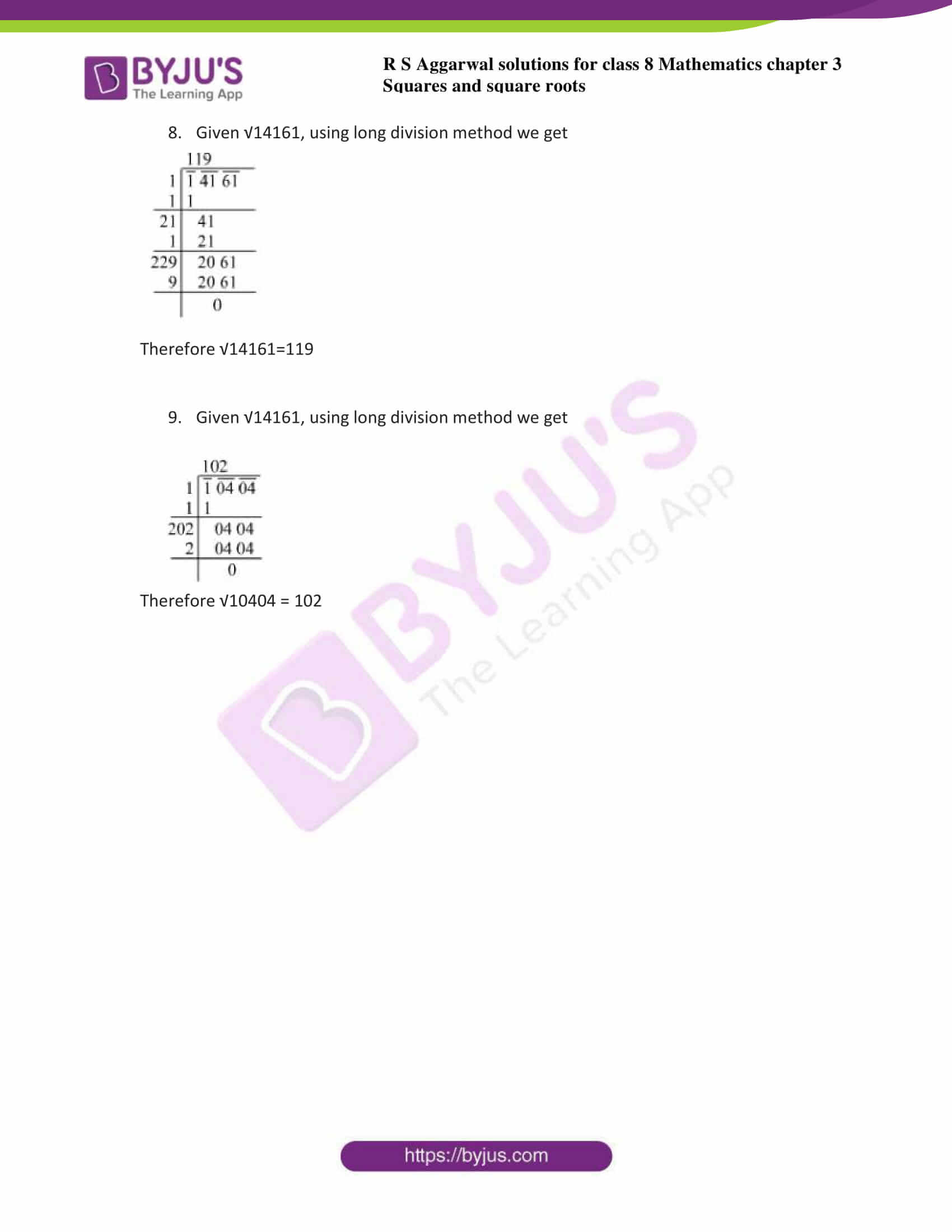### Access answers to Maths RS Aggarwal Solutions for Class 8 Chapter 3 – Squares and Square Roots Exercise 3E

1. Evaluate:

1. √576
2. √1444
3. √4489
4. √6241
5. √7056
6. √9025
7. √11449
8. √14161
9. √10404

Solution: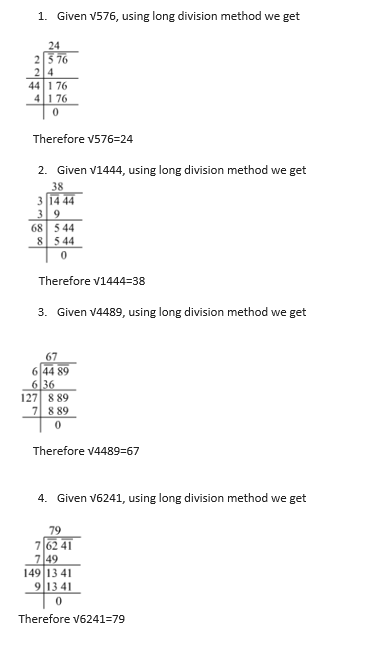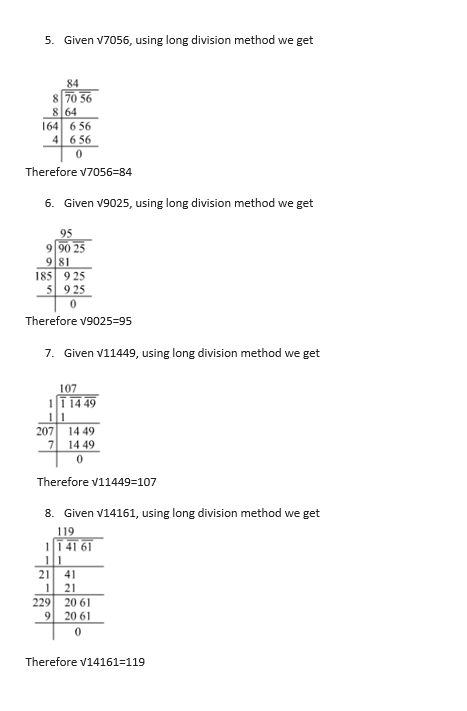9. Given √14161, using long division method we get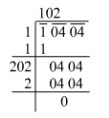Therefore √10404 = 102

### Access other exercises of RS Aggarwal Solutions for Class 8 Maths Chapter 3 – Squares and Square Roots

Exercise 3A Solutions 6 questions

Exercise 3B Solutions 13 questions

Exercise 3C Solutions 8 questions

Exercise 3D Solutions 18 questions

Exercise 3F Solutions 12 questions

Exercise 3G Solutions 10 questions

Exercise 3H Solutions 19 questions

## RS Aggarwal Solutions for Class 8 Maths Chapter 3 – Squares and Square Roots Exercise 3E

Exercise 3E of RS Aggarwal Solutions for Chapter 3, Squares and Square Roots deals with the basic concepts related to the long division method. We can say that this exercise mainly deals with the means for detecting the square root of a perfect square by the long division method that the students have learned in this chapter. Some of the topics focused upon before exercise 3E include the square root of a perfect square by the long division method

The RS Aggarwal Solutions can help the students in practising and learning every concept as it provides solutions to all questions asked in the RS Aggarwal textbook. The RS Aggarwal Solutions are solved in a precise manner easily understandable by the students to clear exams.